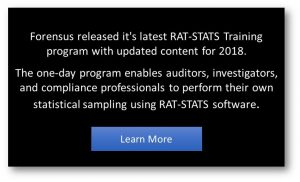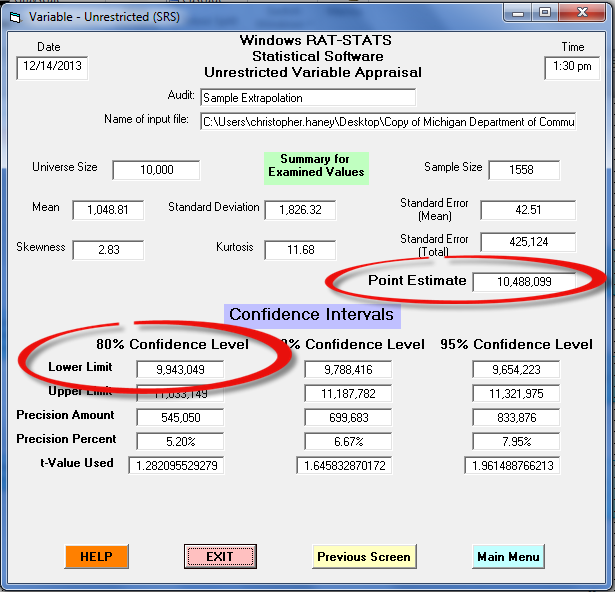Consider the concept of statistical inference (i.e. extrapolation).  Following the proper design, selection and analysis of a sample, we can assume the characteristics witnessed in the sample can be reasonably estimated (i.e. inferred) for the total population … extrapolation expert

## The Output of Extrapolation

The result of this inference is a range of values wherein we expect to find the true value for the population.  Note that a confidence interval, as opposed to a single value, is achieved because the sample is most likely not an exact replica of the total population, and therefore the chances of any single estimate being exactly equal to the true value is infinitesimally small. extrapolation expert

## Point Estimate vs. Lower-BoundWhile the academic conclusion of extrapolation is a range of values, we typically prefer a single estimated value for practical purposes. For instance, auditors seeking to recover overpayments from a provider generally prefer a specific dollar value to estimate overpayments and to simplify recovery efforts, rather than a range of values where the true value is anticipated to lie.  In simple random sampling, for example, a single point-estimate is generally determined. expert

In addition to a point-estimate, another value may be used to yield a single estimated value – the lower-bound of the confidence interval, which it may be preferred in certain analysis since it tends not to over-estimate conclusions.  Evaluating extrapolated results, including the selection of an appropriate value to accomplish an analysis’ objectives, is described further in STEP EIGHT of Forensus’ Sampling Framework.

## Using RAT-STATS for Extrapolation

The mathematical techniques for extrapolating a sample’s conclusions vary depending on the design and methodology of the sample. For example, simple expansion, as described in the preceding paragraph, can be used for simple random sample designs, while different calculations are required for stratified or multistage methodologies.  This text does not delve into these various equations; however, it is critical that analysts ensure the extrapolation method and calculations properly conform to the sample design and methodology.  OIG’s RAT-STATS offers a function specifically designed to help analysts extrapolate the results of sample analysis.  Based upon the results of a sample analysis, RAT-STATS can calculate various useful statistics, including the point-estimate and the lower limit (i.e. lower-bound) for a particular population.  The figure below illustrates an example of RAT-STATS’ extrapolation tool for a hypothetical variable analysis extrapolation.

## Figure: Example RAT-STATS Extrapolation Output## Considerations for Extrapolated Results Extrapolation Expert

Extrapolated conclusions present the user with an actual range of uncertainty (i.e. confidence and precision), while our analysis was designed to achieve a desired level of uncertainty.  This distinction exists since many of our inputs during the Planning and Design phase (i.e. STEPS 1-4 of Forensus’ Sampling Framework) were estimates.  If our estimates were overly conservative, the actual precision of our conclusions might achieve a better-than-desired precision.  Similarly, the actual precision could be poorer-than-desired if our estimates were insufficient.

Therefore, correctly calculating a sample size does not guarantee that a desired level of precision will be achieved.  It is incumbent on the analyst to evaluate the actual precision of the conclusions and to ensure they are sufficiently useful for the purposes of the analysis.  Otherwise, analysis with subpar precision may be deemed to be inadequate. The example output from RAT-STATS in the figure notably calculates the actual precision at various confidence levels, along with multiple estimates, such as the point-estimate and the lower-limit.

Whether statistical software or manual calculations of extrapolation are performed, analysts should ensure each step of the extrapolation analysis is properly documented, along with a record of all statistical software computations before proceeding to STEP EIGHT of Forensus’ Sampling Framework: Evaluating the Results.

## Extrapolation Expert

© Forensus Group, LLC  |  2017